# Mathematical Models

The models shown here were ordered from the German company Martin Schilling in the early 1900s by J E A Steggall, the University's first Professor of Mathematics. Most are plaster but some are wire or paper. They represent the three-dimensional appearance of various mathematical equations - some more complex than others!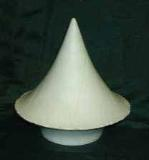Left to right: Surface of rotation of constant negative curvature (conical type) (DUNUC 3082)
Surface of rotation of constant negative curvature (Hyperboloid type) (DUNUC 3125)
Surface of rotation of the Tractrix (Pseudosphere) (DUNUC 3083)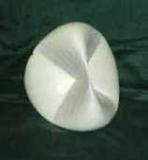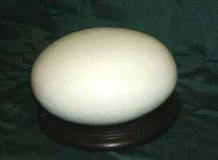Left to right: Surface of rotation of Steiner's Roman Surface (DUNUC 3084)
Sphere (DUNUC 3085)
Ellipsoid (DUNUC 3126)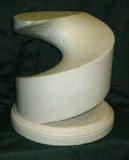Left to right: Unduloid (DUNUC 3086)
Helicoid (DUNUC 3087)
Hyperbolic Paraboloid (DUNUC 3089)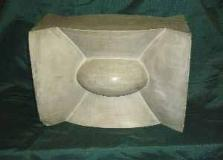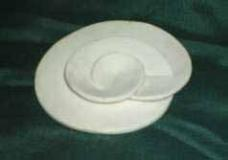Left to right: Catenoid (DUNUC 3090)
Union of Ellipsoid confocal and one Sheeted Hyperboloid (DUNUC 3088)
Riemann surface with branch point of order two (DUNUC 3123)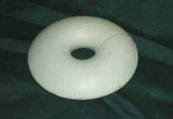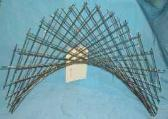Left to right: Triply-connected Riemann surface (DUNUC 3124)
Torus (DUNUC 3832)
Hyperbolic Paraboloid (DUNUC 3096)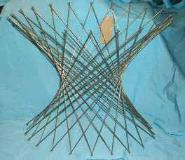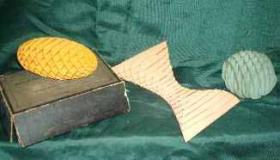Half Hyperboloid (DUNUC 3097)
Hyperboloid of revolution (ellipsoid cross-section) (DUNUC 3100)
Paper models of Surfaces of the Second Order (DUNUC 3074)

Edit